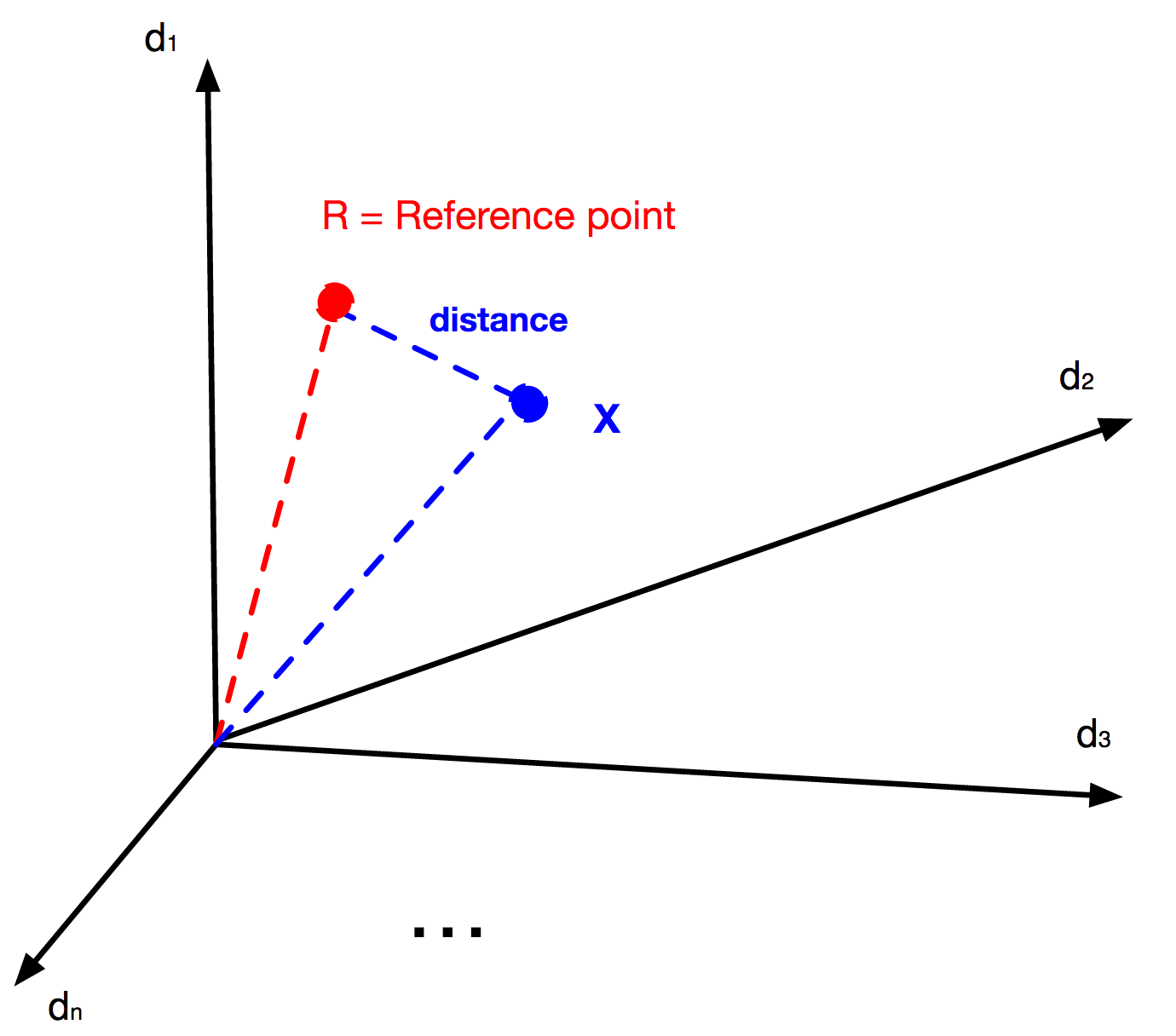## Saturday 22 November 2014

### A simple way of calculating anomaly

Suppose you have a set of n-dimensional variables like the following:

S = { (X1, X2, X3, ..., Xn) | Xi € Di , 1 < i < n }

and we also know somehow that the reference value should be something like the following:

R =  (R1, R2, R3, ..., Rn)

And now we want to now if a Xj is an anomaly or not? Note that you can always assume that your sampled values each with n-dimension can be shown in an n-dimensional euclidean space, even if some dimensions are not numeric. For example if samples are specification of a student in grade 12, like final courses score, weight, height, behaviour, age, number of friends, the skin color and ...  you can always map all of this information for a sampled student to a vector X = (X1, X2, X3, ..., Xn) in an n-dimensional space. You just need some transformation functions to transform non-numeric values to numeric values. In other words for each non-numeric Xi , you need a Ffunction which accepts some non-numeric values in its domain and transforms them to numeric values in its range.You can always bring sampled variable to a n-dimensional euclidean space
OK, so until now we have our sample variable in n-dimensional space, we also suppose that we do have our reference variable which we compare our sampled students with that too.

Now all you have to do is to calculate the distance between the reference point and the sample X. There are many ways to do that, and one of the easiest on is calculating the euclidean distance from the following formula.

D = √ ∑ (Xi - Ri).(Xi - Ri)

After calculating this distance we know how much the sampled variable X is far from the reference variable, and the only thing we need to determine if X is the anomaly or not is a margin for being an anomaly. We will talk about how to define this anomaly margin, for now just accept we can have such a value if we name it M as margin, then the X will be an anomaly if  D > M  and will be normal sample if D ≤ M.

Note that there are many ways to calculate the distance between X and R and each of them has its own pros and cons. You have to get in deep to the problem you are facing with to choose one of this ways.